Ex 10.3

Chapter 10 Class 11 Straight Lines
Serial order wise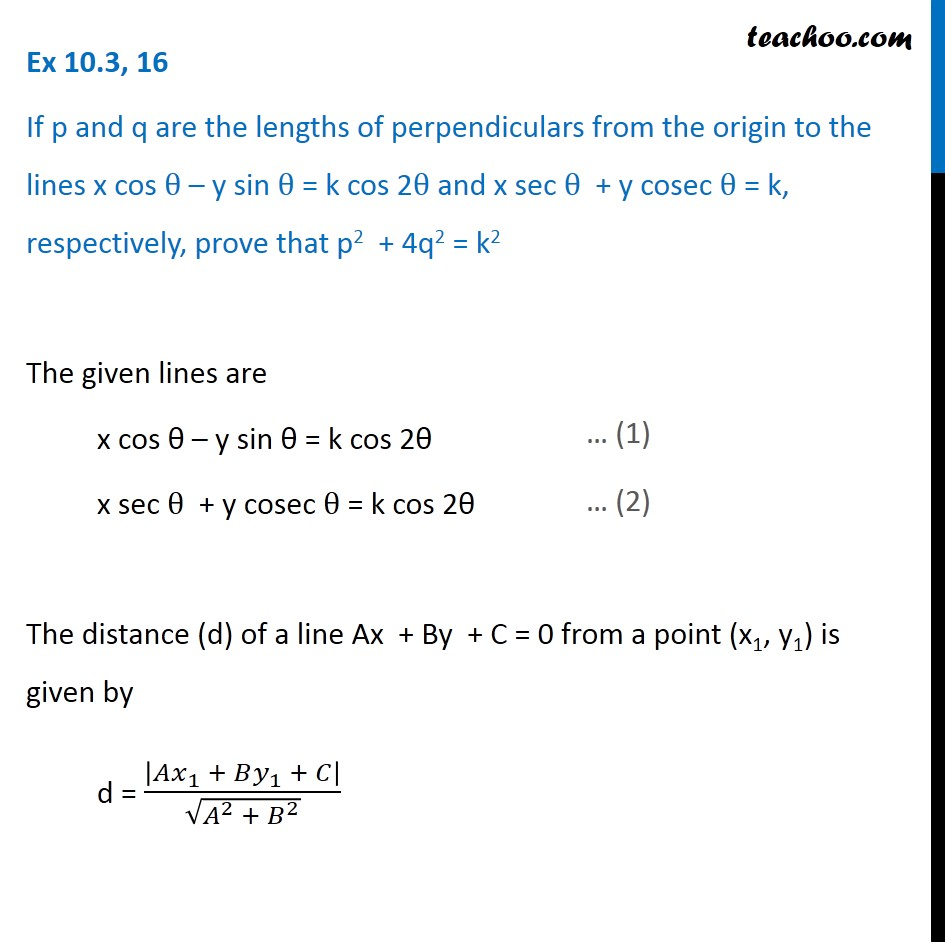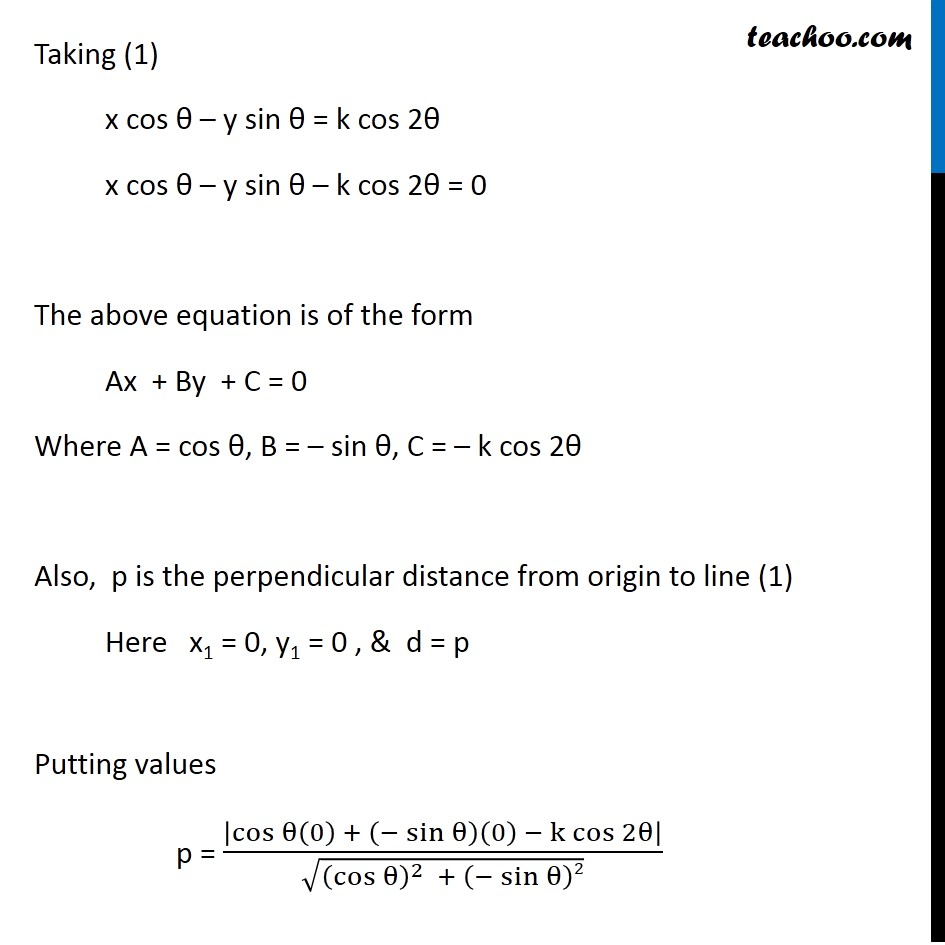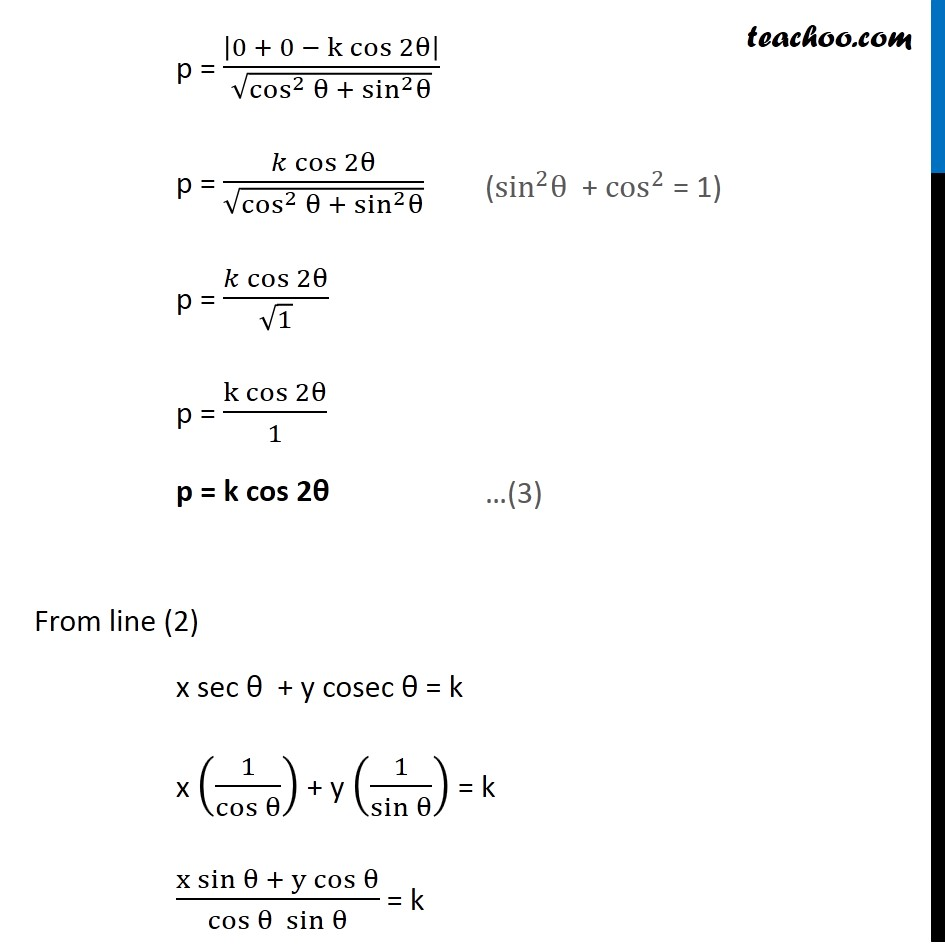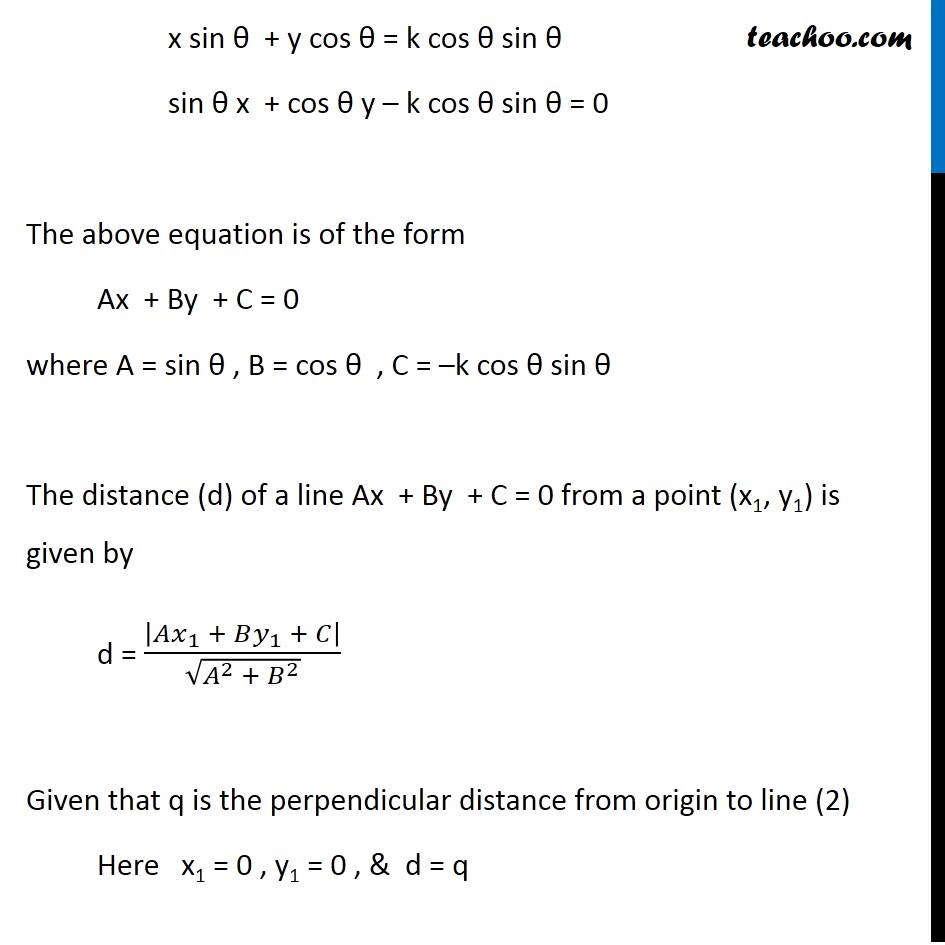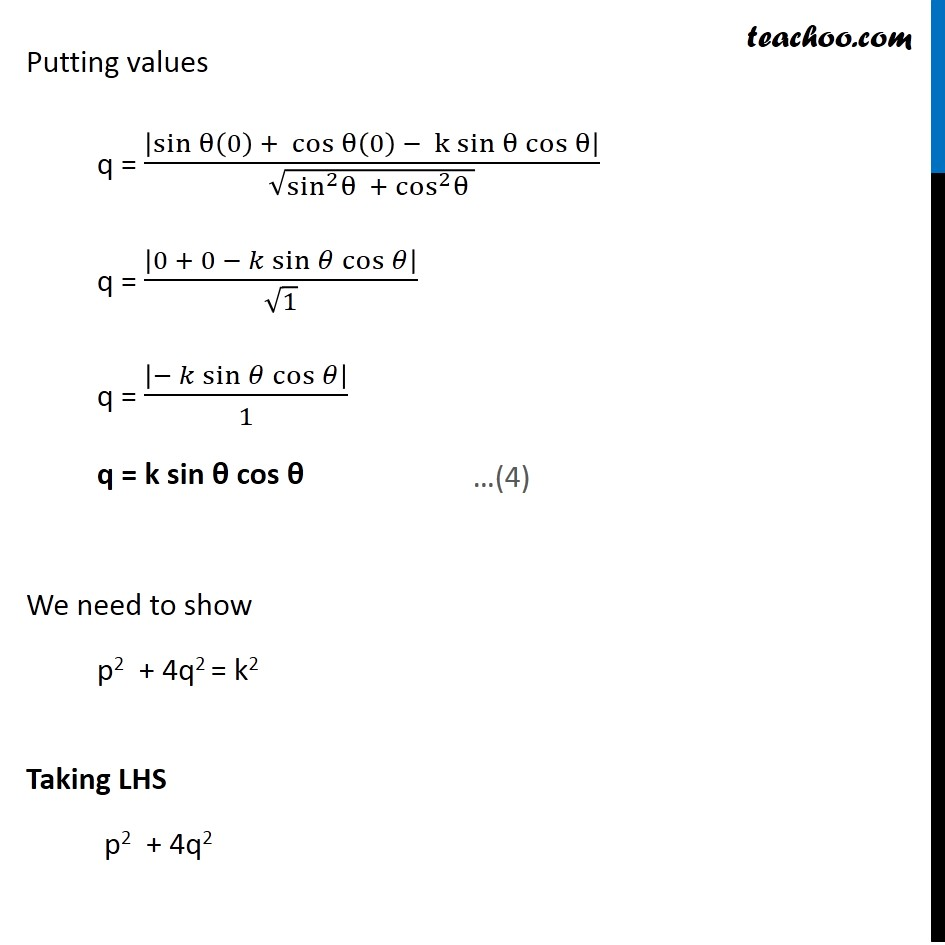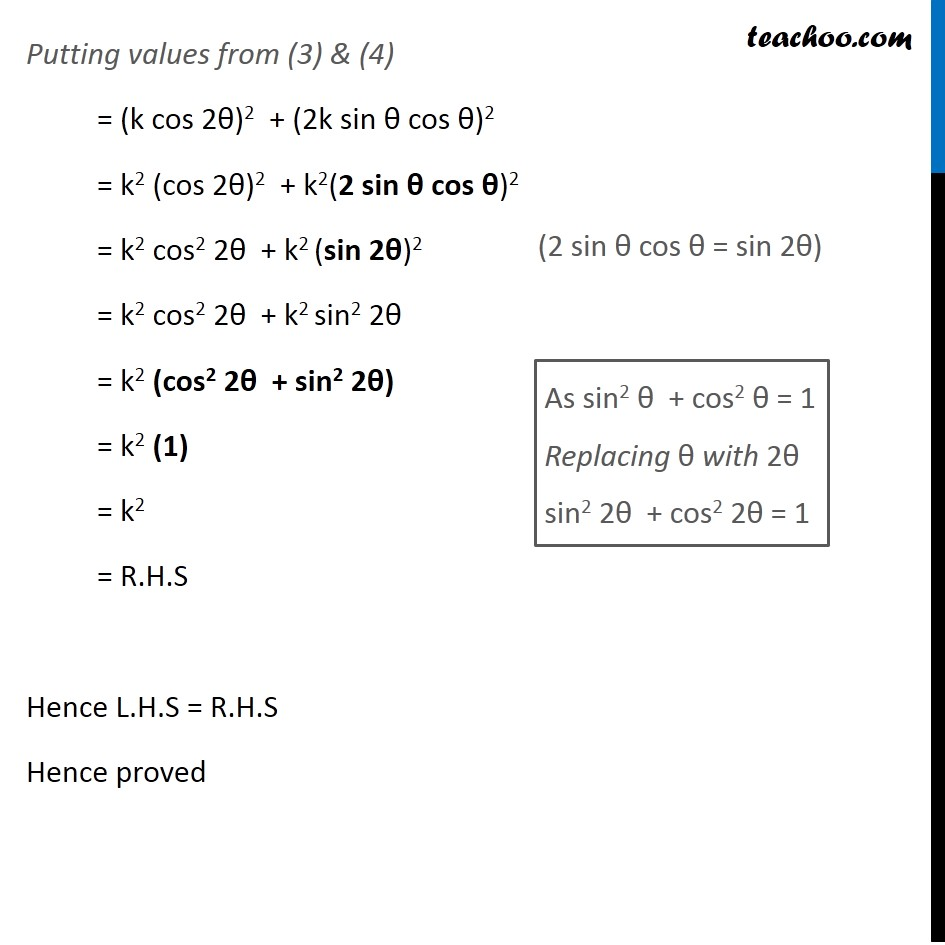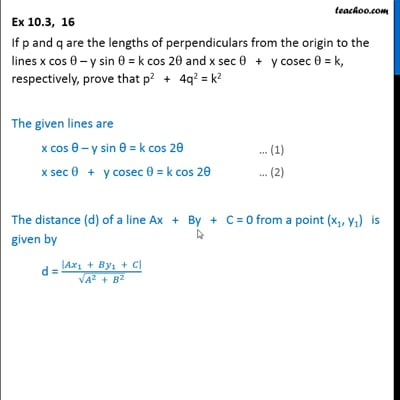This video is only available for Teachoo black users

Introducing your new favourite teacher - Teachoo Black, at only ₹83 per month

### Transcript

Ex 10.3, 16 If p and q are the lengths of perpendiculars from the origin to the lines x cos θ – y sin θ = k cos 2θ and x sec θ + y cosec θ = k, respectively, prove that p2 + 4q2 = k2 The given lines are x cos θ – y sin θ = k cos 2θ x sec θ + y cosec θ = k cos 2θ The distance (d) of a line Ax + By + C = 0 from a point (x1, y1) is given by d = |𝐴𝑥_1 + 𝐵𝑦_1 + 𝐶|/√(𝐴^2 + 𝐵^2 ) Taking (1) x cos θ – y sin θ = k cos 2θ x cos θ – y sin θ – k cos 2θ = 0 The above equation is of the form Ax + By + C = 0 Where A = cos θ, B = – sin θ, C = – k cos 2θ Also, p is the perpendicular distance from origin to line (1) Here x1 = 0, y1 = 0 , & d = p Putting values p = |cos⁡〖θ(0) + (−sin⁡θ )(0) − k cos⁡2θ 〗 |/√((cos⁡θ )^2 + (−sin⁡θ )2) Taking (1) x cos θ – y sin θ = k cos 2θ x cos θ – y sin θ – k cos 2θ = 0 The above equation is of the form Ax + By + C = 0 Where A = cos θ, B = – sin θ, C = – k cos 2θ Also, p is the perpendicular distance from origin to line (1) Here x1 = 0, y1 = 0 , & d = p Putting values p = |cos⁡〖θ(0) + (−sin⁡θ )(0) − k cos⁡2θ 〗 |/√((cos⁡θ )^2 + (−sin⁡θ )2) Taking (1) x cos θ – y sin θ = k cos 2θ x cos θ – y sin θ – k cos 2θ = 0 The above equation is of the form Ax + By + C = 0 Where A = cos θ, B = – sin θ, C = – k cos 2θ Also, p is the perpendicular distance from origin to line (1) Here x1 = 0, y1 = 0 , & d = p Putting values p = |cos⁡〖θ(0) + (−sin⁡θ )(0) − k cos⁡2θ 〗 |/√((cos⁡θ )^2 + (−sin⁡θ )2) x sin θ + y cos θ = k cos θ sin θ sin θ x + cos θ y – k cos θ sin θ = 0 The above equation is of the form Ax + By + C = 0 where A = sin θ , B = cos θ , C = –k cos θ sin θ The distance (d) of a line Ax + By + C = 0 from a point (x1, y1) is given by d = |𝐴𝑥_1 + 𝐵𝑦_1 + 𝐶|/√(𝐴^2 + 𝐵^2 ) Given that q is the perpendicular distance from origin to line (2) Here x1 = 0 , y1 = 0 , & d = q Putting values q = |sin⁡〖θ(0) + 〖 cos〗⁡〖θ(0) − k sin⁡〖θ cos⁡θ 〗 〗 〗 |/√(sin^2 θ + cos^2 θ ) q = |0 + 0 − 𝑘 sin⁡〖𝜃 cos⁡𝜃 〗 |/√1 q = |− 𝑘 sin⁡〖𝜃 cos⁡𝜃 〗 |/1 q = k sin θ cos θ We need to show p2 + 4q2 = k2 Taking LHS p2 + 4q2 Putting values from (3) & (4) = (k cos 2θ)2 + (2k sin θ cos θ)2 = k2 (cos 2θ)2 + k2(2 sin θ cos θ)2 = k2 cos2 2θ + k2 (sin 2θ)2 = k2 cos2 2θ + k2 sin2 2θ = k2 (cos2 2θ + sin2 2θ) = k2 (1) = k2 = R.H.S Hence L.H.S = R.H.S Hence proved As sin2 θ + cos2 θ = 1 Replacing θ with 2θ sin2 2θ + cos2 2θ = 1